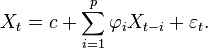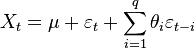Sunday, November 1, 2009

ARMA(p,q) forecasting

ARMA, or Autoregressive Moving Average, offers a relatively simple time series forecasting model. So what about non-stationary financial time series without much autocorrelation, would it perform well?

ARMA(p,q) basics

Forecasting model or process in which both autoregression analysis and moving average methods are applied to a well-behaved time series data. ARMA assumes that the time series is stationary-fluctuates more or less uniformly around a time-invariant mean. Non-stationary series need to be differenced one or more times to achieve stationarity. ARMA models are considered inappropriate for impact analysis or for data that incorporates random 'shocks.' See also autoregressive integrated moving average (ARIMA) model.

Autoregressive Model, AR(p)where are the parameters of the model, c is a constant and e is white noise. The constant term is omitted by many authors for simplicity.

Moving Average Model, MA(q)where the θ1, ..., θq are the parameters of the model, μ is the expectation of Xt (often assumed to equal 0), and the e, e,... are again, white noise error terms.

Source: Wikipedia

Some thoughts off empirical findings

As ARMA was created to address stationary processes, ARMA forecasting resulted much more reliably with percentage returns (of equal length time steps) instead of raw financial time series. Practical application for trading strategies could surface with more analysis of conditional error distributions. Reliability however remains an issue until conditional volatility management. Over all, this simple method presents some promising capabilities!